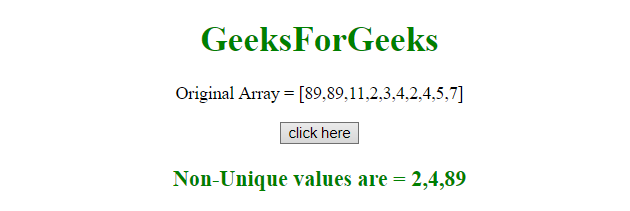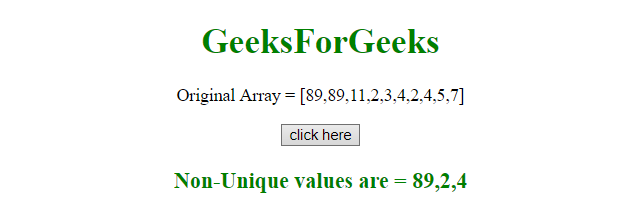# JavaScript | Get all non-unique values from an array

We have a Javascript array and we want to find the all non-unique elements of the array. To get the all non-unique values from the array here are few examples.

Array Slice() method
This method returns the selected elements in a new array object.
This method gets the elements starting from the specified start argument, and ends at excluding the given end argument.
Syntax:

```array.slice(start, end)
```

Parameters:

• start:This parameter is optional. It specifies the integer from where to start the selection (index starts from 0). Negative numbers are used to select from the end of array. If not used, it acts like “0”.
• end:This parameter is optional. It specifies the integer from where the end of the selection. If not used all elements from the start to end of array will be selected. Negative numbers are used to select from the end of array.

Example-1: This example first sort the array and then selects them one by one if they are non-unique.

 ` ` `<``html``> ` ` `  `<``head``> ` `    ``<``title``> ` `        ``JavaScript  ` `      ``| Get all non-unique values. ` `    `` ` ` ` ` `  `<``body` `style``=``"text-align:center;"` `id``=``"body"``> ` `    ``<``h1` `style``=``"color:green;"``>   ` `            ``GeeksForGeeks   ` `        `` ` `    ``<``p` `id``=``"GFG_UP"` `style``=``"font-size: 16px;"``> ` ` `  `    `` ` `    ``<``button` `onclick``=``"gfg_Run()"``> ` `        ``click here ` `    `` ` `    ``<``p` `id``=``"GFG_DOWN"` `style="color:green;  ` `                            ``font-size: 20px;  ` `                            ``font-weight: bold;"> ` `    `` ` `    ``<``script``> ` `        ``var el_up = document.getElementById("GFG_UP"); ` `        ``var el_down = document.getElementById("GFG_DOWN"); ` `        ``var arr = [89, 89, 11, 2, 3, 4, 2, 4, 5, 7]; ` `        ``var sort_arr = arr.slice().sort(); ` `        ``var res = []; ` `        ``el_up.innerHTML = "Original Array = [" + arr + "]";; ` ` `  `        ``function gfg_Run() { ` `            ``for (var i = 0; i < ``sort_arr.length` `- 1; i++) { ` `                ``if (sort_arr[i + 1] == sort_arr[i]) { ` `                    ``res.push(sort_arr[i]); ` `                ``} ` `            ``} ` `            ``el_down.innerHTML` `= ``"Non-Unique values are = "` `+ res; ` `        ``} ` `    `` ` ` ` ` `  ` `

Output:

• Before clicking on the button:• After clicking on the button:Example-2:This example make a dictionary and then store the frequency of each element. Later if they found out to be frequency more than 1, then the element will be selected.

 ` ` `<``html``> ` ` `  `<``head``> ` `    ``<``title``> ` `        ``JavaScript ` `      ``| Get all non-unique values. ` `    `` ` ` ` ` `  `<``body` `style``=``"text-align:center;"` `id``=``"body"``> ` `    ``<``h1` `style``=``"color:green;"``>   ` `            ``GeeksForGeeks   ` `        `` ` `    ``<``p` `id``=``"GFG_UP"` `style``=``"font-size: 16px;"``> ` ` `  `    `` ` `    ``<``button` `onclick``=``"gfg_Run()"``> ` `        ``click here ` `    `` ` `    ``<``p` `id``=``"GFG_DOWN"` `style="color:green;  ` `                            ``font-size: 20px;  ` `                            ``font-weight: bold;"> ` `    `` ` `    ``<``script``> ` `        ``var el_up = document.getElementById("GFG_UP"); ` `        ``var el_down = document.getElementById("GFG_DOWN"); ` `        ``var arr = [89, 89, 11, 2, 3, 4, 2, 4, 5, 7]; ` `        ``el_up.innerHTML = "Original Array = [" + arr + "]";; ` ` `  `        ``function gfg_Run() { ` `            ``var len = arr.length, ` `                ``output = [], ` `                ``count = {}; ` ` `  `            ``for (var i = 0; i < ``len``; i++) { ` `                ``var ``item` `= ``arr``[i]; ` `                ``count[item] = count[item] >= 1 ? count[item] + 1 : 1; ` `                ``if (count[item] === 2) { ` `                    ``output.push(item); ` `                ``} ` `            ``} ` `            ``el_down.innerHTML = "Non-Unique values are = " + output; ` `        ``} ` `    `` ` ` ` ` `  ` `

Output:

• Before clicking on the button:• After clicking on the button:My Personal Notes arrow_drop_upCheck out this Author's contributed articles.

If you like GeeksforGeeks and would like to contribute, you can also write an article using contribute.geeksforgeeks.org or mail your article to contribute@geeksforgeeks.org. See your article appearing on the GeeksforGeeks main page and help other Geeks.

Please Improve this article if you find anything incorrect by clicking on the "Improve Article" button below.

Article Tags :

Be the First to upvote.

Please write to us at contribute@geeksforgeeks.org to report any issue with the above content.